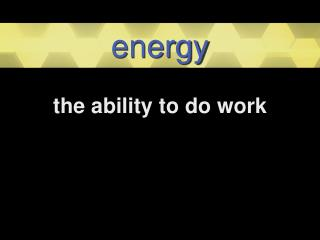DownloadDownload Presentationenergy

# energy

Télécharger la présentation## energy

- - - - - - - - - - - - - - - - - - - - - - - - - - - E N D - - - - - - - - - - - - - - - - - - - - - - - - - - -
##### Presentation Transcript

1. energy the ability to do work

2. potential energy stored energy because of an object’s position kinetic energy energy due to an object’s motion

3. highest potential energy gaining potential energy kinetic energy

4. mechanical energy the ability to make something move

5. motion the change in an object’s position

6. Distance how far an object travels amount of space between 2 points

7. Distance ÷ Time = Speed If you travel 2 miles in 5 minutes: 2 miles ÷ 5 minutes .4 5 2 .0 .4 mi/minute 2 0 0

8. Distance ÷ Time = Speed To change .4 mi/minute to mi/hr: .4 x60 24 mi/hr 24 . 0

9. 100 meters in 5 seconds 20 20 m/sec 100 5 100 0

10. 50 yards in 6 seconds 8 .3 8.3 yd/sec 6 50 48 2 0 18 2

11. instantaneous speed speed of an object at any one moment average speed average speed an object travels during an entire trip

12. Speed x Time = Distance 100 km/h for 3 hours 100 300 kilometers x 3 300

13. velocity distance an object moves over a given amount of time in a certain direction

14. Dad is driving west at 105 km/h. west 105 km/h velocity

15. Jim runs 10 km/h. 10 km/h not velocity

16. velocity scientific common used interchangeably with speed, leaving out direction as a factor distance an object moves over a given amount of time in a certain direction

17. acceleration scientific common to go faster a change in velocity (change in speed and/or direction)

18. deceleration scientific common to slow down a negative change in velocity (change opposite of acceleration)

19. Lindy maintains the 150 mi/h speed as she travels north on the track. velocity

20. Rodney comes alongside and matches Lindy’s speed. Lindy increases her speed to get to the finish line before Rodney. acceleration

21. force a push or pull applied to an object in order to change its velocity

22. friction a force that keeps objects from moving against other objects

24. 1st Law of Motion An object tends to stay at the same velocity unless another force causes it to change.

25. inertia resistance to a change in motion

26. gravity the pull of one object on another object

27. 2nd Law of Motion Force is equal to an object’s mass and the acceleration of the object. mass x acceleration = force

28. Principles the greater the mass of the object, the greater the force needed to move it the greater the force, the greater the acceleration the greater the acceleration, the greater the force on the object

29. mass x acceleration = force ma = f force ÷ mass = acceleration f/m = a force ÷ acceleration = mass f/a = m

30. 3rd Law of Motion When one object exerts a force on another object, the second object reacts by exerting an equal force back on the first object. Law of action and reaction

31. https://edvance360.com/v7/c_video_player.php?c=10&video=2657

32. work a force acting on something and moving it a distance

33. force x distance = work If an object does not move, the distance is zero. any number x 0= Therefore, no work is done. 0

34. force x distance = work newtons × meters = joules

35. force x distance = work You use 4 newtons of force to lift a box 3 meters: 4 newtons x 3 meters 12 joules

36. force x distance = work You use 6 newtons of force to lift a box 2 meters: 6 newtons x 2 meters 12 joules The same amount of work is done!

37. machine any object that makes work easier

38. simple machines lever pulley wheel and axle inclined plane wedge screw

39. Simple Machines increase speed increase force change the direction of the force

40. Machines can’t reduce the amount of work; they just make work easier. force x distance = work

41. lever any bar that turns on a point seesaw wheelbarrow broom

42. effort force force applied to simple machine resistance force force that works against the effort

43. First Class Lever effort resistance fulcrum

44. First-class lever resistance effort fulcrum

45. Second-class lever resistance effort fulcrum

46. Third-class lever effort resistance fulcrum

47. pulley grooved wheel with a chain or rope wrapped in the groove

48. fixed pulley attached to something that does not move